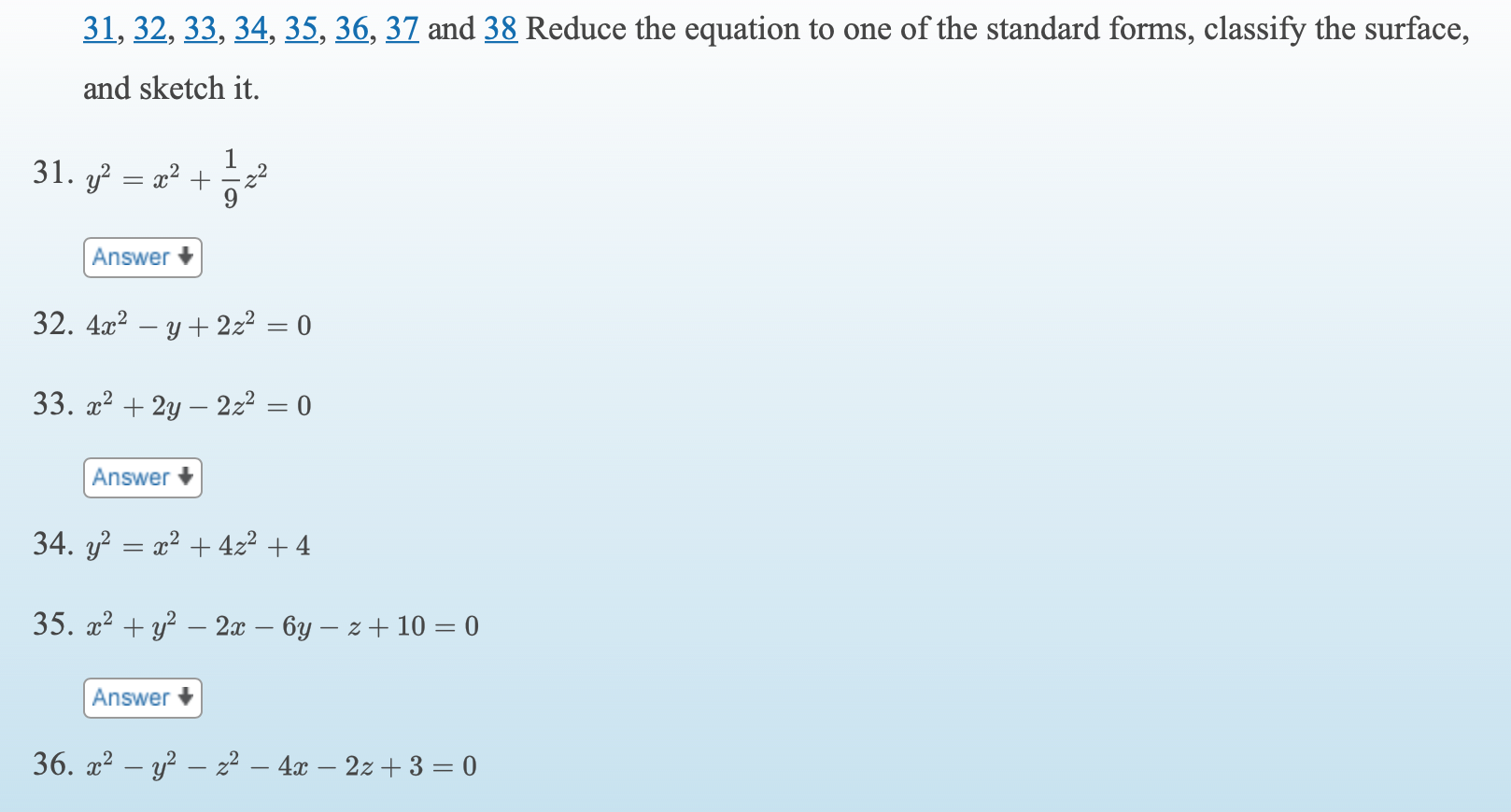# 31, 32, 33, 34, 35, 36, 37 and 38 Reduce the equation to one of the standard forms, classify the surface,and sketch it.31. yx2 +Answer +32. 4x? – y + 2z2 = 033. x2 + 2y – 2z2 = 0Answer34. y? = x2 + 422 + 435. a? + y? – 2x – 6y – z + 10 = 0Answer +36. x2 – y² – z² – 4x – 2z + 3 = 0

Question
4 views

I just need #36.help_outlineImage Transcriptionclose31, 32, 33, 34, 35, 36, 37 and 38 Reduce the equation to one of the standard forms, classify the surface, and sketch it. 31. y x2 + Answer + 32. 4x? – y + 2z2 = 0 33. x2 + 2y – 2z2 = 0 Answer 34. y? = x2 + 422 + 4 35. a? + y? – 2x – 6y – z + 10 = 0 Answer + 36. x2 – y² – z² – 4x – 2z + 3 = 0 fullscreen
check_circle

Step 1

Given the equation

Step 2

Write the equation in t...

### Want to see the full answer?

See Solution

#### Want to see this answer and more?

Solutions are written by subject experts who are available 24/7. Questions are typically answered within 1 hour.*

See Solution
*Response times may vary by subject and question.
Tagged in

### Calculus